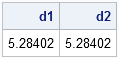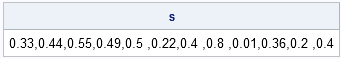Over at the SAS/IML Discussion Forum, there have been several posts about how to call a Base SAS functions from SAS/IML when the Base SAS function supports a variable number of arguments.

It is easy to call a Base SAS function from SAS/IML software when the syntax for the function is a fixed number of parameters: simply use the same syntax in SAS/IML as you use for the DATA step.

The problem arises for functions that support a varying number of parameters. One solution uses a slick trick that I developed and put in my book, Statistical Programming with SAS/IML Software.

### What's the Problem?

The problem is that some Base SAS functions such as the DUR function, FINANCE function, and NPV function (in general, a lot of the financial functions) have a syntax that supports a varying number of arguments. The Base SAS function expects a comma-separated list of parameters, whereas in SAS/IML it is natural to store the parameters in a vector.

How can you convert a vector of values into a comma-separated list? The trick is to do the following:

1. Convert the vector of values into a comma-delimited string.
2. Put the string into a macro variable by using the SYMPUT function.
3. Use the macro variable in the Base SAS function.

### A Canonical Example

The syntax for the DUR function supports an arbitrary number of parameters:

d = dur(Y, Freq, c1, ..., ck);
The last k parameters are usually specified either by a comma-delimited list or by using an array and the OF operator, as shown in the following example:

```data Duration; /** first approach: use comma-delimited list **/ d1 = dur(1/20,1,.33,.44,.55,.49,.5,.22,.4,.8,.01,.36,.2,.4); /** alternative approach: use array **/ Y = 1/20; freq = 1; array C (.33,.44,.55,.49,.50,.22,.4,.8,.01,.36,.2,.4); d2 = dur(Y, freq, of C[*]); run;   proc print noobs; var d1 d2; run;```However, when you try to do the "natural" thing in SAS/IML software, you get an error:

```proc iml; use Duration; read all var {Y freq}; vNames = "c1":"c12"; read all var vNames into C; close Duration;   /** OF syntax is not supported in SAS/IML **/ d = dur(Y, freq, of C); /** ERROR: doesn't work! **/```

The problem is that the OF syntax is not supported in SAS/IML software. The DUR function in Base SAS is expecting a comma-separated list of arguments, not a SAS/IML vector.

### Macro to the Rescue: Converting a Vector into a Comma-Separated String

The following statements define a SAS/IML module called GetArgList that takes a vector of values and creates a comma-delimited string from it. The module uses the PUTN function to convert from numbers to character values, and uses the ROWCAT function to form a single string:

```/** convert numeric elements to a single string **/ start GetArgList(v); y = strip(putn(rowvec(v),"BEST12.")) + ","; /** get rid of last comma **/ y[ncol(y)] = strip(putn(v[ncol(y)],"BEST12.")); return( rowcat(y) ); /** scalar string **/ finish;   s = GetArgList(C); print s;```This function is what we need to call the DUR function (and similar functions) with parameters that are contained in a SAS/IML vector. The trick is to save the comma-delimited string, s, into a macro variable, and then call the DUR function with that macro variable:

```call symput("myArgs", s); /** create macro var **/ d = dur(Y, freq, &myArgs); /** use macro var **/```

Although it is a bit of a hack, this technique enables you to call Base SAS functions that have a varying number of parameters, and pass in values from a SAS/IML vector.

Share

### About AuthorDistinguished Researcher in Computational Statistics

Rick Wicklin, PhD, is a distinguished researcher in computational statistics at SAS and is a principal developer of PROC IML and SAS/IML Studio. His areas of expertise include computational statistics, simulation, statistical graphics, and modern methods in statistical data analysis. Rick is author of the books Statistical Programming with SAS/IML Software and Simulating Data with SAS.

### 8 Comments

1.how to convert a character variable in to numeric within proc IML

•Use the NUM function.

2.In SAS9.4 ,can write this . Funny thing ?

NOTE: IML Ready
57 Y = 1/20;
58 freq = 1;
59 C={.33,.44,.55,.49,.50,.22,.4,.8,.01,.36,.2,.4};
60 d = dur(Y, freq, C);
61 print d;
62 quit;
NOTE: Exiting IML.

The result is wrong. Very funny . IML didn't complain it .

•The result is not wrong. The result follows from the rules for calling Base functions from IML The syntax you've shown is equivalent to calling the DUR function with three arguments and changing the third argument for each call. The equivalent DATA step statements are
d = dur(Y, freq, .33); output;
d = dur(Y, freq, .44); output;
d = dur(Y, freq, .55); output;
...
d = dur(Y, freq, .4; output;

3.Prenil Sewmohan on

Hi, thank you for the useful post. I have one additional question here, I was wondering if there is a way to convert a comma separated string of numbers that have been defined as a character string into a vector of numeric type numbers?

•Yes. Use the Base SAS COUNTC function to count the delimiters (=commas) and use the SCAN function to break the string into words. Then use the SAS/IML NUM function to convert the characters to numbers:

```proc iml; s = "1, 23,456,7,89, 9"; numCommas = countc(s, ","); numbers = num( scan(s, 1:numCommas) );```